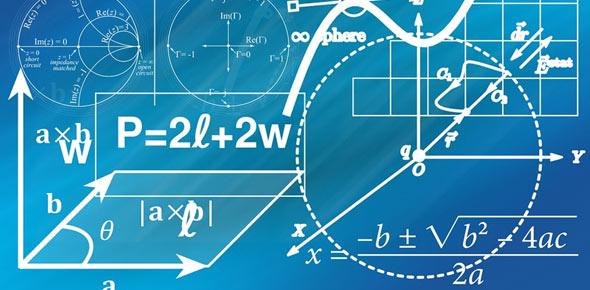# Distance Formula

7 Questions | Total Attempts: 290SettingsDistance FormulaVOISE Academy Algebra Ms. Johnson

Related Topics
• 1.
Find the distance between (2,2) and (6,2).
• 2.
Find the distance between (1,4) and (6,4).
• 3.
Find the distance between (2,-3) and (4,-3).
• 4.
Find the distance between (-1,3) and (2,-1).
• 5.
Find the distance between (-3,-2) and (5,4).
• 6.
Find the distance between (-6,-3) and (6,6).
• 7.
Find the distance between (-8,2) and (4,7).